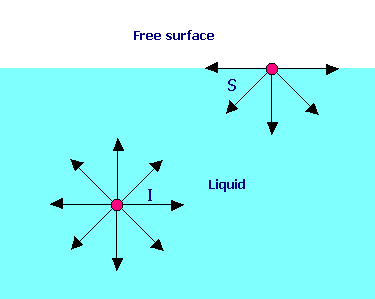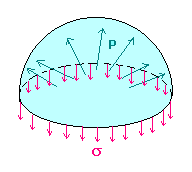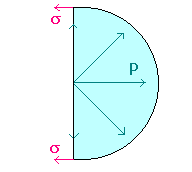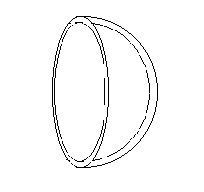### Surface Tension

Home -> Lecture Notes -> Fluid Mechanics -> Unit-IA molecule I in the interior of a liquid is under attractive forces in all directions and the vector sum of these forces is zero. But a molecule S at the surface of a liquid is acted by a net inward cohesive force that is perpendicular to the surface. Hence it requires work to move molecules to the surface against this opposing force, and surface molecules have more energy than interior ones.

The surface tension (s sigma) of a liquid is the work that must be done to bring enough molecules from inside the liquid to the surface to form one new unit area of that surface (J/m2 = N/m). Historically surface tensions have been reported in handbooks in dynes per centimeter (1 dyn/cm = 0.001 N/m).

Surface tension is the tendency of the surface of a liquid to behave like a stretched elastic membrane. There is a natural tendency for liquids to minimize their surface area. For this reason, drops of liquid tend to take a spherical shape in order to minimize surface area. For such a small droplet, surface tension will cause an increase of internal pressure p in order to balance the surface force.We will find the amount D (Dp = p - poutside) by which the pressure inside a liquid droplet of radius r, exceeds the pressure of the surrounding vapor/air by making force balances on a hemispherical drop. Observe that the internal pressure p is trying to blow apart the two hemispheres, whereas the surface tension s is trying to pull them together. Therefore, Dp pr2 = 2prs
i.e. Dp = 2s/rSimilar force balances can be made for cylindrical liquid jet.
Dp 2r= 2s
i.e. Dp = s/rSimilar treatment can be made for a soap bubble which is having two free surfaces. Dp pr2 = 2 x 2prs
i.e. Dp = 4s/r

Surface tension generally appears only in situations involving either free surfaces (liquid/gas or liquid/solid boundaries) or interfaces (liquid/liquid boundaries); in the latter case, it is usually called the interfacial tension.

Representative values for the surface tensions of liquids at 20oC, in contact either with air or their vapor (there is usually little difference between the two), are given in Table.

 Liquid Surface Tension s dyne/cm Benzene 23.70 Benzene 28.85 Ethanol 22.75 Glycerol 63.40 Mercury 435.50 Methanol 22.61 n-Octane 21.78 Water 72.75